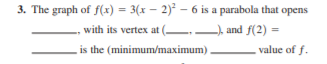# 3. The graph of f(x) = 3(x – 2)² – 6 is a parabola that opens with its vertex at ( ), and f(2) = is the (minimum/maximum) value of f.

Question

The graph of f1x2  31x  22
2  6 is a parabola that opens
, with its vertex at 1 , 2, and f122 
is the (minimum/maximum) value of f.help_outlineImage Transcriptionclose3. The graph of f(x) = 3(x – 2)² – 6 is a parabola that opens with its vertex at ( ), and f(2) = is the (minimum/maximum) value of f. fullscreen

### Want to see this answer and more?

Experts are waiting 24/7 to provide step-by-step solutions in as fast as 30 minutes!*

*Response times may vary by subject and question complexity. Median response time is 34 minutes for paid subscribers and may be longer for promotional offers.
Tagged in
Math
Algebra

### Other